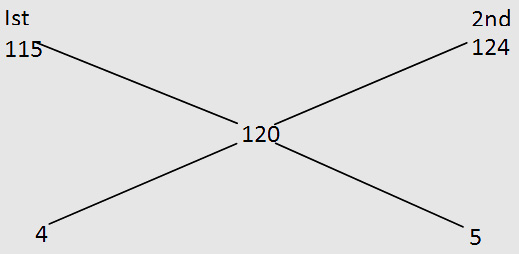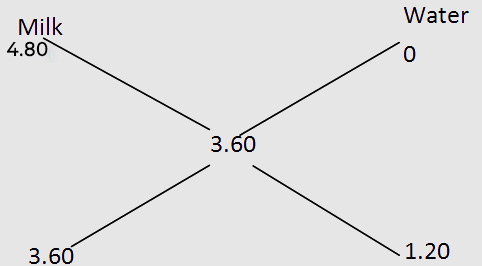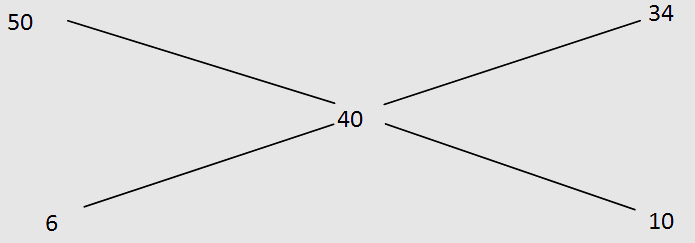# Quantitative Aptitude Quiz for SSC (Alligation and Mixture): 28 April 2021

Updated Wed, 28 Apr 2021 05:21 PM ISTSource: safalta
Q.1. In what ratio must two kinds of sugar at Rs. 1.15 and Rs. 1.24 per kg be mixed so that by selling at Rs. 1.50 per kg, 25% may be gained?

### Free Demo Classes

1. 4: 5
2. 5: 4
3. 1: 1
4. 2: 3

Sol : Option 1
SP = 1.50 Rs. Profit = 25%. CP = 1.50 x (100/125) = 1.20Rs.Therefore required ratio = 4 : 5

Q.2. How much water be mixed in 36 litre of milk worth Rs. 5.00 per litre, so that value of mixture is Rs. 3.60 per litre?

1. 10 litres
2. 12 litres
3. 11 litres
4. 14 litres

Sol : Option 2Ratio of Milk to water will be= 18:6,
In 18 litre milk water added = 6 litre
In 1 litre milk water added = 6 / 18
In 36 litre milk water added = (6/18) x 36 = 12 litres

Q.3. How many kg of Sugar at 50 P per kg must a man mix with 25 kg of sugar at 34P per kg so that by selling the mixture at 44P per kg he gains 10% on the outlay?

1. 10 kg
2. 15 kg
3. 20 kg
4. 16 kg

Sol : Option 2
Cost price of mixture = (44/110) x 100 = 40∴ sugar of 50 paisa per kg to be mixed = (3/5) x 25 = 15kg

Q.4. How much sugar at Rs. 9.5 a kg should be added to 17 kg of tea at Rs. 20 a kg so that the mixture be worth Rs. 13 a kg.?

1. 11 kg
2. 17 kg
3. 21 kg
4. 34 kg

Sol : Option 4
Ratio in which tea and sugar should be mixed
= 20 – 13 : 13 – 9.5 = 7 : 3.5 ⇒ 7 : 3.5 ⇒ 2:1 .
Let x be quantity at 9.5/kg. ∴ 2 : 1 = x : 17,hence x = 34 kg.

Q.5. A hospital uses a mixture of salt and water at Rs. 7.62/litre. This mixture contains 5 % salt. Another mixture containing 75 % water costs Rs. 7.82/litre. How much does the patient pay if he buys 5 litres of mixture containing 18% salt?

1. Rs. 83.75
2. Rs. 73.85
3. Rs. 37.85
4. Rs. 38.75

Sol : Option 4
1st mixture contains 5 % salt. 2nd mixture contains 75 % water i.e. 25 % salt.
Required % of salt = 18 %. ∴ Required ratio = 25 – 18 : 18 – 5 = 7 : 13 and required price of the mixture = 7.82 – x : x – 7.62 = 7 : 13 ⇒ x = 7.75/ liter. Hence price of 5 liters of this mixture = 7.75 x 5 = Rs. 38.75.

Q.6. How many kg of custard powder costing Rs. 42 per kg must be mixed with 16 kg of custard powder costing Rs. 60 per kg so that 20 % may be gained by selling the mixture at Rs. 60 per kg?

1. 11 kg
2. 14 kg
3. 12 kg
4. 20 kg

Sol : Option 4
SP = 60. Gain= 20%. CP = (100/120) x 60. ∴ CP = 50.
Ratio between the 2 varieties of custard powder = 60 – 50 : 50 –42 = 10 : 8. ∴ if x is the required quantity then 10 : 8 = x : 16 ⇒ x =20 kg.

Q.7. A vessel is full of mixture of spirit and water in which there is 20 per cent of spirit. 5 litres are drawn off and the vessel is filled up with water. If the spirit is now 12 %, find the total quantity in the vessel (in ltrs).

1. 72
2. 12.5
3. 60
4. 7.2

Sol : Option 2
Let the total mixture be x liters.
It contains 20 % spirit i.e.0.2 of the total mixture. When 5 liters are drawn off, quantity of spirit removed = 0.2(5) = 1 liters.
Remaining spirit = 0.2x –1.
Also remaining spirit = 0.12x. ∴ 0.2x - 1 = 0.12x ⇒ x = 12.5L.

Q8. How much chicory at Rs. 5 a kg should be added to 20 kg of coffee at Rs. 12 a kg so that the mixture be worth Rs. 7.50 a kg.?

1. 21 kg
2. 15 kg
3. 36 kg
4. 42 kg

Sol : Option 3
Ratio in which coffee and chicory should be mixed
= 12 – 7.5 : 7.5 – 5 = 4.5 : 2.5 ⇒ 9 : 5.
Let x be quantity at 4/kg. ∴ 9 : 5 = x : 20 ⇒ x = 36kg.

Q9. An alloy of copper and nickel contains 65 % copper. A second alloy contains copper and nickel in the ratio 17 : 3. In what ratio should the two alloys be mixed so that the new mixture contains 4 times as much copper as nickel?
1. 4 : 5
2. 5 : 4
3. 1 : 3
4. 2 : 3

Sol : Option 3
First alloy – 65% copper. ∴ 35% Nickel. Ratio is 65 : 35
⇒ 13 : 7. 2nd alloy – Ratio is 17 : 3 ∴ Proportion of copper in the 1st alloy = 13/20 and proportion of copper in the 2nd alloy = 17/20.
Also proportion of copper in resulting alloy = 4/5. Hence required ratio is (17/20 – 4/5) : (4/5 – 13/20) = 1 : 3.

Q10. Five litres of wine is removed from a cask full of wine and is replaced with water. Five litres of this mixture is then removed and replaced with water. If the ratio of wine to water in the cask is now 16 : 9, how much wine did the cask hold ?

1. 25 litres
2. 50 litres
3. 100 litres
4. 150 litres

Sol : Option 1
Let the cask holds x liters of wine. 5 liters of wine is replaced with water.
This operation is done 2 times. ∴ [(x - 5) / x]2 = 16 / (16+9)
⇒ [(x - 5) / x]2 = 16 / 25
⇒ [(x - 5) / x] = 4/5 ⇒ x = 25 liters

### Disclaimer

अपनी वेबसाइट पर हम डाटा संग्रह टूल्स, जैसे की कुकीज के माध्यम से आपकी जानकारी एकत्र करते हैं ताकि आपको बेहतर अनुभव प्रदान कर सकें, वेबसाइट के ट्रैफिक का विश्लेषण कर सकें, कॉन्टेंट व्यक्तिगत तरीके से पेश कर सकें और हमारे पार्टनर्स, जैसे की Google, और सोशल मीडिया साइट्स, जैसे की Facebook, के साथ लक्षित विज्ञापन पेश करने के लिए उपयोग कर सकें। साथ ही, अगर आप साइन-अप करते हैं, तो हम आपका ईमेल पता, फोन नंबर और अन्य विवरण पूरी तरह सुरक्षित तरीके से स्टोर करते हैं। आप कुकीज नीति पृष्ठ से अपनी कुकीज हटा सकते है और रजिस्टर्ड यूजर अपने प्रोफाइल पेज से अपना व्यक्तिगत डाटा हटा या एक्सपोर्ट कर सकते हैं। हमारी Cookies Policy, Privacy Policy और Terms & Conditions के बारे में पढ़ें और अपनी सहमति देने के लिए Agree पर क्लिक करें।

Agree

Get free counselling session